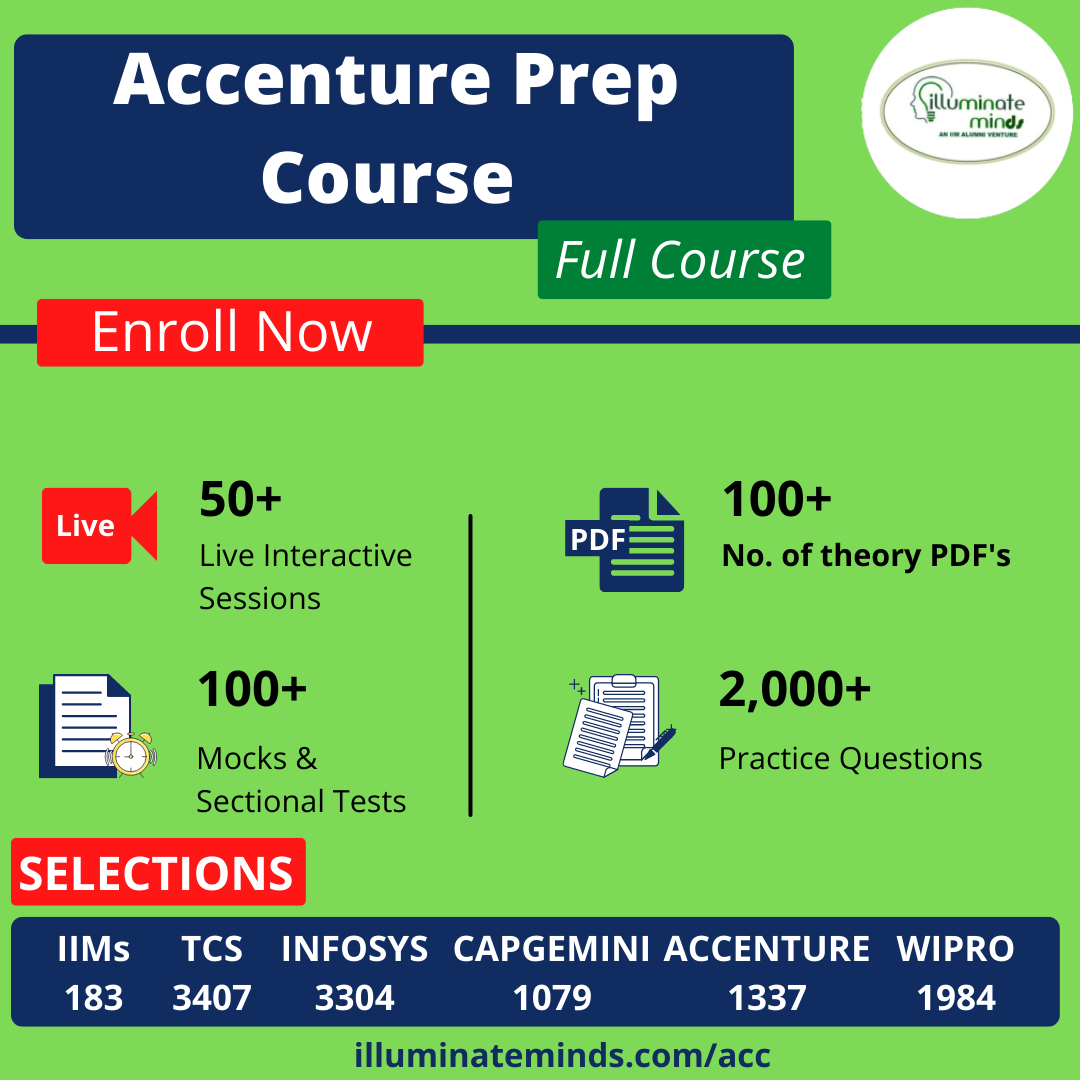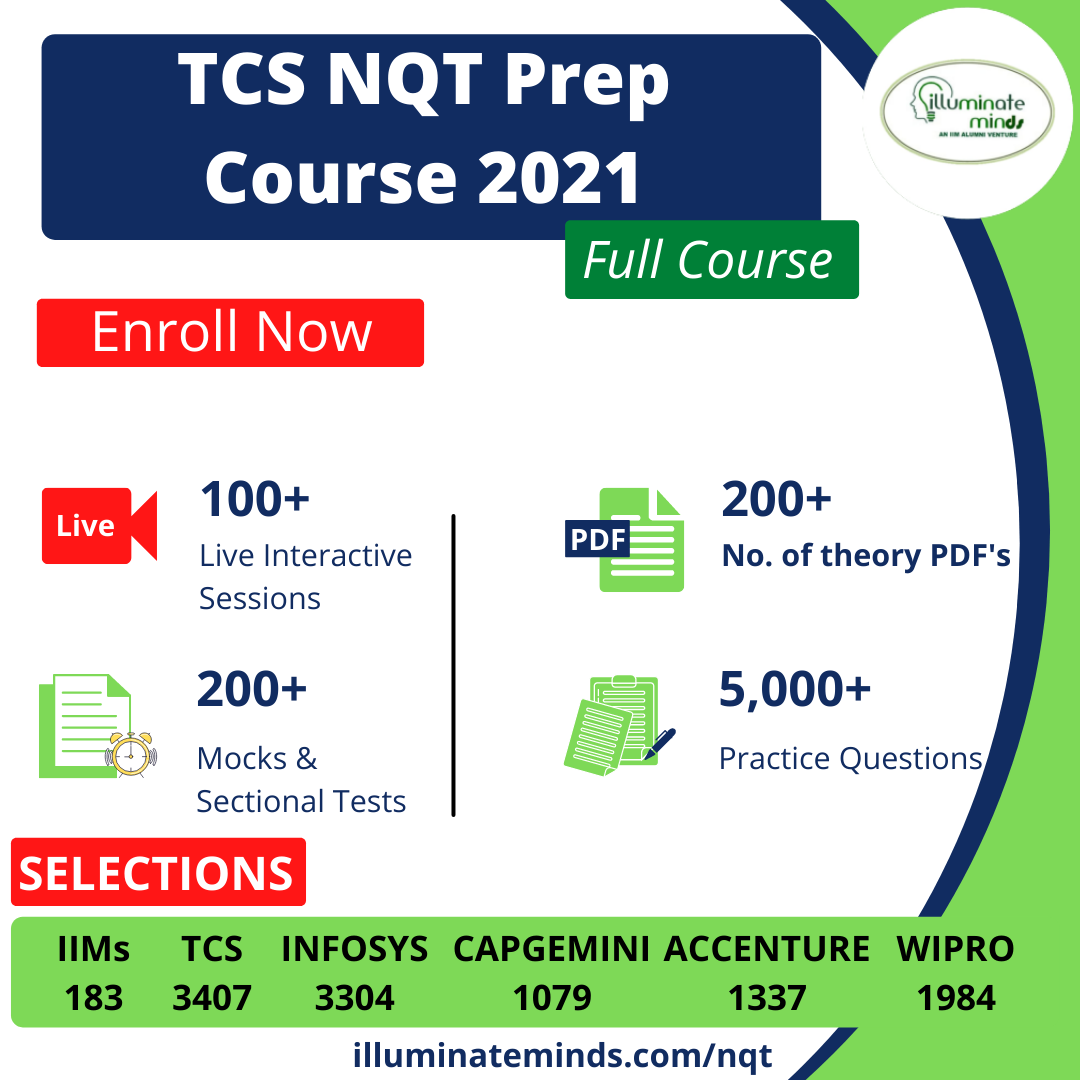CAT | Coordinate-Geometry

## CAT | Coordinate-GeometryJobs By Batch
Jobs By Location
Jobs By Degree
Jobs By Branch
IT Jobs
Internships
Govt. Jobs• ##### Zoho off campus drive 2022/ 2023 | Multiple Hirings | Any Graduates/ Post Graduates, BE, B.Tech, MBA, MCA, ME, M.Tech | 2019, 2020, 2021, 2022, 2023 Batch | Multiple Location• ##### Original Questions of IT Companies| All Topics### Quiz Begins Here

Q #1
:

#### CAT Coordinate Geometry: Integer coordinates

Set S contains points whose abscissa and ordinate are both natural numbers. Point P, an element in set S has the property that the sum of the distances from point P to the point (3,0) and the point (0,5) is the lowest among all elements in set S. What is the sum of abscissa and ordinate of point P?

+

Q #2
:

#### CAT Coordinate Geometry: Geometric Probability

Region R is defined as the region in the first quadrant satisfying the condition 3x + 4y < 12. Given that a point P with coordinates (r, s) lies within the region R, what is the probability that r > 2?

+

Q #3
:

#### CAT Coordinate Geometry: Greatest integer function

Region Q is defined by the equation 2x + y < 40. How many points (r, s) exist such that r is a natural number and s is a multiple of r?

+

Q #4
:

#### CAT Coordinate Geometry: Points equidistant from lines

What is the equation of a set of points equidistant from the lines y = 5 and x = �4?

+

Q #5
:

#### CAT Coordinate Geometry: Area under curve

What is the area enclosed in the region defined by y = |x � 1| + 2, line x = 1, X�axis and Y�axis?

+